### Cool interactive tool for C++

My husband actually directed me to this awesome tool. I have always loved python’s interactive notebooks and wished more languages had their own version. It was so easy to run demos and workshops in a browser and really helped the learning process. Well now there is an interactive workflow for C++ from Jupyter!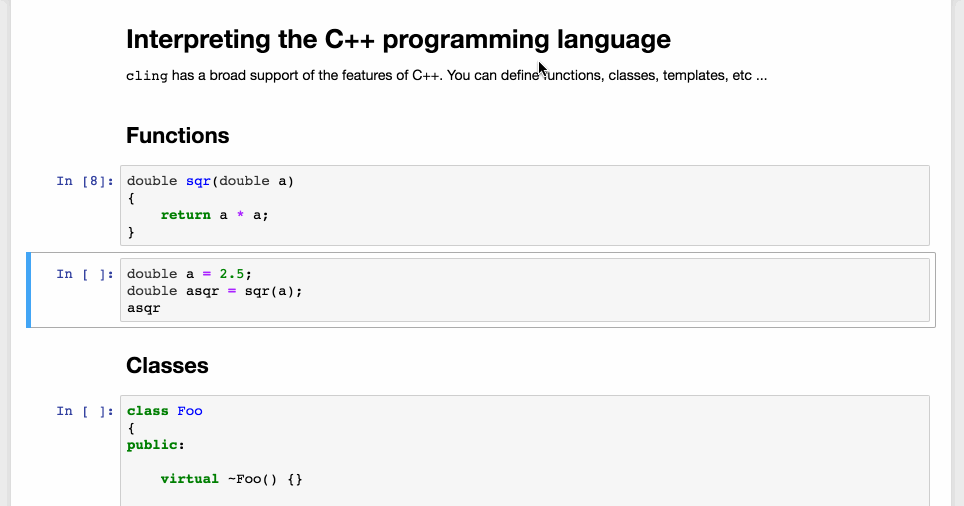### Weighted Edge Graph Data Structure in C++

So this data structure is one of the more complicated structures I’ll post about, I think I’ll be done with C++ for awhile.

A brief description of a graph, as explained by Wikipedia, “A graph data structure consists of a finite (and possibly mutable) set of vertices or nodes or points, together with a set of unordered pairs of these vertices for an undirected graph or a set of ordered pairs for a directed graph. These pairs are known as edges, arcs, or lines for an undirected graph and as arrows, directed edges, directed arcs, or directed lines for a directed graph. The vertices may be part of the graph structure, or may be external entities represented by integer indices or references.”

This being said, in simple, it is a structure with connected node points. An ideal use for it, is determining the shortest path. Take on the airplane analogy. What is the shortest path an airlines can put you through? There are so many ways to fly from one place to another, think of all the possible transfers, not just a direct flight. So a graph data structure would be perfect for storing each airport and the total distance to each one. Then when a customer comes to buy a ticket, it will be easy to calculate the shortest path for them to their destination.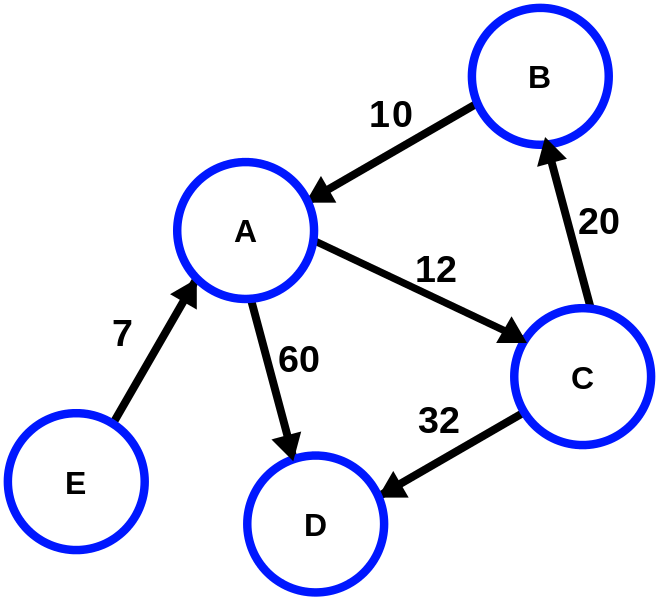Just imagine A, B, C, D, E are different airports and the numbers show the distance to each

We are specifically going to look at graphs with weighted edges, from our analogy above, the weighted edge describes the distance to each airport. The weight determines which route the algorithm will take, ideally the lesser weight because it will be the shortest path.

```#include <iostream>
#include <vector>
#include <assert.h>

using namespace std;

class graph{
public:
static const size_t MAX = 20;

graph(){
numVerticies = 0;
edges = new int*[MAX];
labels = new char*[MAX];
for(size_t i = 0; i < MAX; i++){
edges[i] = new int[MAX];
labels[i] = nullptr;
}
for (size_t i = 0; i < MAX; i++){
for(size_t j = 0; j < MAX; j++) {
edges[i][j] = -1;
}
}
}

~graph() {
delete[] labels;
delete[] edges;
}

void add_edge(int source, int target, int weight=0) {
assert(source >= 0 && source < numVerticies);
assert(target >= 0 && target < numVerticies);

edges[source][target] = weight;
}

assert(numVerticies < MAX);
for(size_t i = 0; i < numVerticies + 1; i++) {
edges[i][numVerticies] = -1;
edges[numVerticies][i] = -1;
}
labels[numVerticies] = new char[strlen(vertexLabel) + 1];
strcpy(labels[numVerticies], vertexLabel);
numVerticies++;
}

void shortestPath(int start) {
int* distance = new int[numVerticies];
bool* marked = new bool[numVerticies];
for(size_t i = 0; i < numVerticies; i++){
marked[i] = false;
}
vector<int> path;
reset(distance);
distance[start] = 0;

cout << "Starting Dijkstra’s Shortest-Distance Algorithm with vertex " << labels[start] << endl;

int min = start;
while(path.size() < numVerticies) {
for (int v = 0; v < numVerticies; v++) {
if (!marked[v]){
if(distance[v] < distance[min]) {
min = v;
}
}
}
//Print it out

if (path.size() > 0){
int src = min;
int src_index = path.size() - 1;
while (edges[path[src_index]][min] != (distance[min] - distance[path[src_index]])){
src_index--;
}
src = path[src_index];
cout << "Vertex Pair " << labels[src] << "," << labels[min] << " ";
cout << "     Pair Cost: " << (distance[min] - distance[src]);
cout << "     Cost From Start: " << distance[min] << endl;
}

path.push_back(min);
marked[min] = true;

for (int i = 0; i < numVerticies; i++) {
if(is_edge(min, i) && (distance[i] > (distance[min] + edges[min][i]))) {
distance[i] = edges[min][i] + distance[min];
}
}
for (int x = 0; x < numVerticies; x++) {
if (!marked[x]){
min = x;
break;
}
}
}

delete[] marked;
delete[] distance;
}

void reset (int *&dist) {
for (int i = 0; i < numVerticies; i++) {
dist[i] = 999999999;
}
}

void DFS(int start) {
assert(start >= 0 && start < numVerticies);

bool* marked = new bool[numVerticies];
for(size_t i = 0; i < numVerticies; i++){
marked[i] = false;
}

cout << "Starting DFS with vertex " << labels[start] << endl;

DFS(start, marked);

cout << endl;

delete[] marked;
}

private:
int** edges;
char** labels;
int numVerticies;

bool is_edge(int source, int target) {
assert(source >= 0 && source < numVerticies);
assert(target >= 0 && target < numVerticies);

return edges[source][target] > -1;
}

void DFS(int start, bool marked[]){
assert(start >= 0 && start < numVerticies);

marked[start] = true;

cout << labels[start] << " ";

for (int i = 0; i < numVerticies; ++i) {
if(edges[start][i] > -1 && !marked[i]) {
DFS(i, marked);
}
}
}
};
```

Good luck and happy programming!

### Structure of a Program

Here is a short read to refresh on how to best structure a C++ program.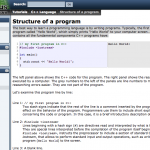Structure of a program – C++ Tutorials The best way to learn a programming language is by writing programs. Typically, the first program beginners write is a program called “Hello World”, which simply prints “Hello World” to your computer screen. Although it is very simple, it contains all the fundamental components C++ programs have: Source: www.cplusplus.com/doc/tutorial/program_structure/

### Simple AVL Tree in C++

An AVL tree is a binary search tree(BST) however, unlike binary search trees, an AVL tree (named after Georgy Adelson-Velsky and Evgenii Landis) is self balancing. So no matter how many nodes you insert into the tree, it will adjust it’s branches and ensure the tree is balanced at all times. Making sure the subtree heights only differ by at most 1. BSTs are great for segregating and storing data with a O(log n) search time. Downside with BST is that it can get weighted on one side and doesn’t have an restrictions to prevent it from getting skewed. By switching to an AVL, data is balanced in the tree and the search time is decreased to log n.

So it is more efficient in most cases to use the AVL tree, below is an example of how to code this in C++. Note that the AVL tree uses a lot of the same code the BST did from this post.

```#pragma once

#include <iomanip>
#include <iostream>

using namespace std;

class AVL
{
public:
AVL(){
root = nullptr;
}
~AVL(){
destroy(root);
}

//My Node class for storing data, note how I add height
struct Node{
int data;
Node *left;
Node *right;
int height;

Node(int d){
data = d;
left = nullptr;
right = nullptr;
height = 0;
}

void updateHeight(){
int lHeight = 0;
int rHeight = 0;
if (left != nullptr) {
lHeight = left->height;
}
if (right != nullptr) {
rHeight = right->height;
}
int max = (lHeight > rHeight) ? lHeight : rHeight;
height = max + 1;
}

};

void insert(int val){
insert(val, root);
}

//Rotate a Node branch to the left, in order to balance things
Node* rotateLeft(Node *&leaf){
Node* temp = leaf->right;
leaf->right = temp->left;
temp->left = leaf;

//update the Nodes new height
leaf->updateHeight();

return temp;
}

//Rotate a Node branch to the right, in order to balance things
Node* rotateRight(Node *&leaf){
Node* temp = leaf->left;
leaf->left = temp->right;
temp->right = leaf;

//update the Nodes new height
leaf->updateHeight();

return temp;
}

//Rotate a Node branch to the right then the left, in order to balance things
Node* rotateRightLeft(Node *&leaf){
Node* temp = leaf->right;
leaf->right = rotateRight(temp);
return rotateLeft(leaf);
}

//Rotate a Node branch to the left then the right, in order to balance things
Node* rotateLeftRight(Node *&leaf){
Node* temp = leaf->left;
leaf->left = rotateLeft(temp);
return rotateRight(leaf);
}

//Function that checks each Node's left and right branches to determine if they are unbalanced
//If they are, we rotate the branches
void rebalance(Node *&leaf){
int hDiff = getDiff(leaf);
if (hDiff > 1){
if (getDiff(leaf->left) > 0) {
leaf = rotateRight(leaf);
} else {
leaf = rotateLeftRight(leaf);
}
} else if(hDiff < -1) {
if (getDiff(leaf->right) < 0) {
leaf = rotateLeft(leaf);
} else {
leaf = rotateRightLeft(leaf);
}
}
}

private:
Node *root;
//Insert a Node (very similar to BST, except we need to update Node height and then check for rebalance)
void insert(int d, Node *&leaf){
if (leaf == nullptr){
leaf = new Node(d);
leaf->updateHeight();
}
else {
if (d < leaf->data){
insert(d, leaf->left);
leaf->updateHeight();
rebalance(leaf);
}
else{
insert(d, leaf->right);
leaf->updateHeight();
rebalance(leaf);
}
}
}

//Same as BST
void destroy(Node *&leaf){
if (leaf != nullptr){
destroy(leaf->left);
destroy(leaf->right);
delete leaf;
}
}

//Get the difference between Node right and left branch heights, if it returns positive
//We know the left side is greater, if negative, we know the right side is greater
int getDiff(Node *leaf){
int lHeight = 0;
int rHeight = 0;
if (leaf->left != nullptr) {
lHeight =  leaf->left->height;
}
if (leaf->right != nullptr) {
rHeight = leaf->right->height
}
return lHeight - rHeight;
}
};```

Let me know if you have any issues!

Here is a short insert on how to remove all Nodes of X value from a list. I use recursion and I believe I’ve created a pretty clean solution. This requires Nodes found in my earlier post.

```#include "Node.h"

//Run the search and remove on the linked list starting at the head
//target is the value we are trying to remove from our list
void searchAndRemove(int target){
}

void searchAndRemove(int target, Node*& n){
if(n != nullptr){
if(n->data == target) { //if we found our target
Node* temp = n;
n = n->next; //delete and replace
delete temp; //delete the node to clean up memory
searchAndRemove(target, n); //continue the search, we need this to check the one used to replace
} else {
searchAndRemove(target, n->next); //continue the search
}
}
}```

Let me know if you have any comments or improvements!

### Binary Search Tree Traversals in C++

So this will pretty much wrap up our short expedition into binary trees. Remember that these functions rely on my earlier posts dealing with the class and nodes.

The three types of traversals we will look at are:

• Preorder (root, left, right)
• Inorder (left, root, right)
• Postorder (left, right, root)

#### Preorder Traversal

This type of traversal can be used to create duplicates of a tree and can be used to implement prefixes. Whatever order of values you put into a tree will be the order that preorder traversal gives you. So If I enter, 5 7 4 2 1 9 8 3 6 into a binary tree, when I use preorder traversal, I will expect to see 5 7 4 2 1 9 8 3 6.

```void preorderTraversal (Node* n) {
if (n != nullptr) { //make sure we have a value
cout << n->data << endl; //Print out the current Node value
preorderTraversal(n->left); //traverse down the left side
preorderTraversal(n->right); //Once we return from the left, go down the right
}
}```

#### Inorder Traversal

This type of traversal will return data sorted in order. So if I entered, 5 7 4 2 1 9 8 3 6, I would expect to see 1 2 3 4 5 6 7 8 9.

```  void inorderTraversal (Node* n) {
if (n != nullptr) { //make sure we have a value
inorderTraversal(n->left); //traverse down the left side
cout << n->data << endl; //Print out the current Node value
inorderTraversal(n->right); //Once we return from the print, go down the right
}
}```

#### Postorder Traversal

This type of traversal can be used to implement postfixes and can cleanly be used to destroy a tree with out leaving Nodes floating around corrupting memory. So if I entered, 5 7 4 2 1 9 8 3 6, I would expect to see 1 3 2 4 6 8 9 7 5

``` void postorderTraversal (Node* n) {
if (n != nullptr) { //make sure we have a value
postorderTraversal(n->left); //traverse down the left side
postorderTraversal(n->right); //Once we return from the left, go down the right
cout << n->data << endl; //Print out the current Node value
}
}```

For more information on binary trees, I would checkout this article. It goes into detail on how traversals on called on the tree and gives some great visuals. I also liked this interactive visual for binary search trees in general.

Good luck!

### Sweet Binary Search Tree Print in C++

I found the basis of a print on Stackover flow the other day and changed it a little bit to be more compatible across compiler versions.

Happy Coding!

```void print() {
}
```

For some reason my code editor wouldn’t do the left slashes so below is a screenshot.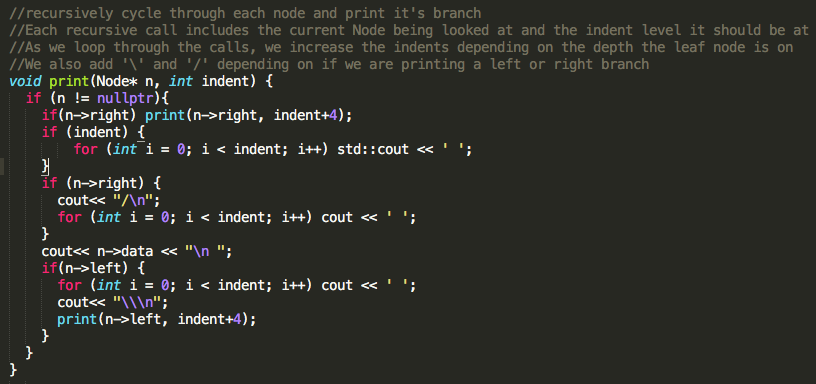Here is an example of what it will look like for the sequence 5 7 4 2 1 9 8 3 6: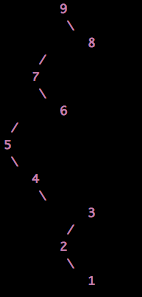### Binary Search Trees! Simple Class in C++

Here is a really basic binary tree class, it just includes the basics of creating, inserting, erasing, and returning size. In later posts I will talk about printing and traversals.

This class also uses the Node.h talked about in this earlier post. You’ll notice that I really like to use recursion, I think this is cleaner than looping.

```#include <assert.h>
#include "Node.h"
using namespace std;

class Bst
{
public:

//constructor for when a head Node is provided and when it is not
Bst() {
root = nullptr;
}

Bst(Node *np) {
root = np;
}

//destroy the tree, we need to go through and destroy each node
~Bst() {
destroyTree(root);
}

//get the number of nodes in the tree
int size() {
return size(root);
}

//erase a value in the tree
void erase(int item) {
erase(item, root);
}

//insert a Node in the tree
void insert(int item) {
insert(item, root);
}

private:

Node* root;

//Go through each branch and recursively destroy all Nodes
void destroyTree(Node*& n) {
if (n != nullptr) {
destroyTree(n->left);
destroyTree(n->right);
delete n;
}
}

//For each Node return the number of left and right nodes
//Add it up recursively to get the total size
int size(Node* n) {
if (n != nullptr) {
int left = size(n->left);
int right = size(n->right);
int self = 1;
return left + self + right;
}
return 0;
}

//Find the minimum Node value
Node* findMin(Node* n){
assert(n != nullptr);
if (n->left != nullptr) {
return findMin(n->left);
}
return n;
}

//this one is a beast
//look through all the nodes recursively
//once you find the node value there are numerous cases we need to look for
//If the current node does not have left and right nodes, just delete it
//If it does have a left or right node, set the child to the parent
//If it has both left and right, we need to work some magic. First we find
//the smallest value and set the node we want to delete to that value (removing it)
void erase(int item, Node*& n) {
if (n != nullptr) {
if (item == n->data) {
if (n->right == nullptr && n->left == nullptr) {
delete n;
n = nullptr;
} else if (n->right == nullptr) {
Node* temp = n;
n = n->left;
delete n;
} else if (n->left == nullptr){
Node* temp = n;
n = n->right;
delete n;
} else {
Node *temp = findMin(n->right);
n->data = temp->data;
erase(item, n->right);
}
} else if (item < n->data) {
erase(item, n->left);
} else {
erase(item, n->right);
}
}
}

//look through all the nodes
//insert the node on the correct node, it will be added to the left if the value is less
//added to the right if the value is greater
void insert(int item, Node*& n) {
if (n != nullptr) {
if (item < n->data) {
insert(item, n->left);
} else {
insert(item, n->right);
}
} else {
n = new Node(item);
}
}
};
```

Let me know if you have any improvements or comments!

### Reading a File with C++

Here is just a simple example of reading a file with iostream. Just follow my comments to understand.

Good luck, sometimes newlines and spaces can mess you up, you may need to utilize an ignore() or something else.

```#include <fstream>
#include <iostream>
using namespace std;

void evaluateFile(string ins); //Do something with the file contents

int main(){
return EXIT_SUCCESS;
}

ifstream input;
string fileName;
cout << "What is the full path of file to be read" << endl;
getline(cin, fileName); //Prompt user for filename, needs to be the fill path
input.open(fileName); //Open thhe file
if (input.fail()){ //Fail case
cout << "Sorry, cannot open file. Quitting now." << endl;
exit(0);
}
while(!input.eof()){ //Read file until the end of file is reached
string line;
getline(input, line);
evaluateFile(line);
}
input.close();
}

void evaluateFile(string line){
cout << line << endl;
//DO SOMETHING WITH THE FILE INPUT
}```

### Setting a timer in C++

I wanted to time some functions to measure performance of different types of functions in order to find what action was optimal. Here is my code for measuring the time of a C++ action.

Basically, you collect a start clock() and a end clock() then find the total time it took to perform something by taking the difference between the two. By default the clock() function uses the CLOCKS_PER_SEC variable to convert the found clocks to seconds. My code shows how to get the result in seconds and Microseconds.

Happy Coding!

```#include <time.h> //Need this for clock()

int main() {
int seconds_to_micro = 1000000; //conversion from seconds to microseconds var

clock_t start = clock(); //Get starting point clock

//***********PERFORM AN ACTION HERE********

clock_t end = clock(); //Get ending point clock

float time_in_seconds = static_cast<float>(end - start)/ CLOCKS_PER_SEC //in seconds
float time_in_microseconds = static_cast<float>(end - start)/ CLOCKS_PER_SEC * seconds_to_micro; //in microseconds

return;
}```